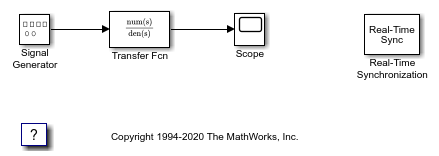# Create a Real-Time Application

A Simulink® model is a graphical representation of your physical system. You create a Simulink model for a non-real-time simulation of your system, and then you use the Simulink model to create a real-time application.

### Open the Model

This example uses `sldrtex_model`. To open this model, in the MATLAB® Command Window, type:

```open_system('sldrtex_model') ```Note: You cannot run a Simulink® Desktop Real-Time® model in rapid accelerator mode.

### Run the Model

To run `sldrtex_model` in Connected IO mode:

To run sldrtex_model as a real-time application:

### Close the Model

To close the model, in the MATLAB Command Window, type:

```bdclose("all"); ```